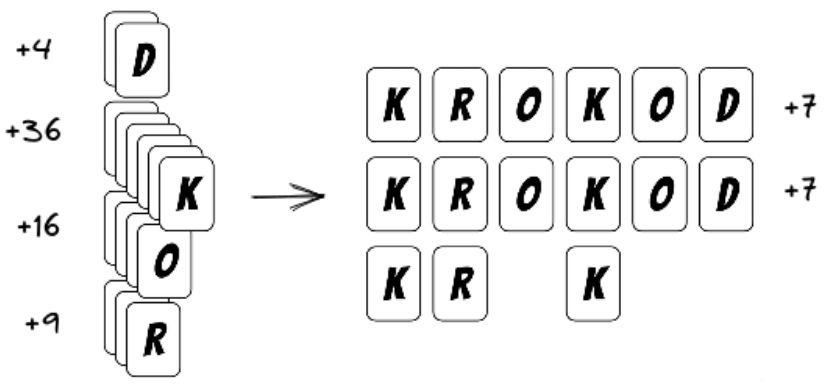시간 제한메모리 제한제출정답맞힌 사람정답 비율
1 초 1024 MB222100.000%

## 문제

When he is not making videos for his YouTube channel, the famous programmer influencer Krokod likes to play boardgames with his friend Paula. He wants to play the game 7Krokods, but Paula doesn’t like complex games, so Krokod decided that they will play only with green and crocodile cards.

Paula has $n$ green cards, and each of them has one of the following letters written on it: d, k, o, or r. Her total score is defined as the sum of the following components:

• For each letter, she gets as much points as is the number of cards with that letter written on them, squared. For example, if she has $6$ cards with the letter k, she gets $36$ points.
• For each word krokod she can make from her cards, she gets an extra $7$ points.

Illustration of the first example.Paula has $2$ letters d ($2 · 2 = 4$ points), $6$ letters k ($6 · 6 = 36$ points), $4$ letters o ($4 · 4 = 16$ points) and $3$ letters r ($3 · 3 = 9$ points). The word krokod can be spelled $2$ times ($7 · 2 = 14$ points). Her total score is $79$. ($4 + 36 + 16 + 9 + 14 = 79$)

Paula also has $m$ crocodile cards. She can replace each of the crocodile cards with a green card having a letter of her choice. She will do it in a way that maximizes her score.

Help her determine the maximum score she can get with her cards.

## 입력

The first line contains integers $n$ and $m$ ($0 ≤ n ≤ 100$, $0 ≤ m ≤ 10$), the number of green cards and the number of crocodile’s cards.

The second line contains a sequence of $n$ characters, where the $i$-th characters represents the letter on the i-th green card. The sequence consists only of characters d, k, o and r.

## 출력

In the first and only line output Paula’s maximum possible score.

## 서브태스크

번호배점제한
117

$m = 0$

226

$m = 1$

37

## 예제 입력 1

15 0
krokodkrokodkrk


## 예제 출력 1

79


## 예제 입력 2

5 1
rokod


## 예제 출력 2

17


## 예제 입력 3

8 2
ddkkoorr


## 예제 출력 3

35


## 힌트

Clarification of the first example: Look at the illustation in the task statement.

Clarification of the second example: For the maximum possible score, Paula can replace her crocodile card with a green card having the letter k.

## 채점 및 기타 정보

• 예제는 채점하지 않는다.